# Circles-NCERT Solutions

Class X Math
NCERT Solution for Circle
NCERT TEXTBOOK QUESTIONS SOLVED
EXERCISE 10.1
Q.1.   How many tangents can a circle have?
Sol. A circle can have an infinite number of tangents.
Q.2.   Fill in the blanks:
(i) A tangent to a circle intersects it in ........... point(s).
(ii) A line intersecting a circle in two points is called a........... .
(iii) A circle can have...........parallel tangents at the most.
(iv) The common point of a tangent to a circle and the circle is called ........... .
Sol. (i) exactly one        (ii) secant        (iii) two        (iv) point of contact.
Q.3.   A tangent PQ at a point P of a circle of radius 5 cm meets a line through the centre O at a point Q so that OQ = 12 cm. Length of PQ is:
(A) 12 cm
(B) 13 cm
(C) 8.5
cm (D)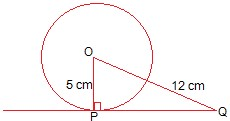Q.4.   Draw a circle and two lines parallel to a given line such that one is a tangent and the other a secant to the circle.
Sol. We have the required figure.
Here, l is the given line and a circle with centre 0 is drawn.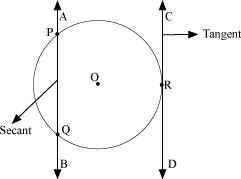The line PT is drawn which is parallel to l and tangent to the circle.
Also, AB is drawn parallel to line l and is a secant to the circle.
EXERCISE 10.2
Q.1.   Choose the correct option:
From a point Q, the length of the tangent to a circle is 24 cm and the distance of Q from the centre is 25 cm. The radius of the circle is
(A) 7 cm
(B) 12 cm
(C) 15 cm
(D) 24.5 cm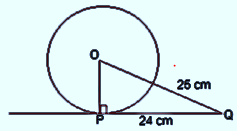Sol. QT is a tangent to the circle at T and OT is
Also, OQ = 25 cm and QT = 24 cm
∴ Using Pythagoras theorem, we get
OQ2 = QT2 + OT2
⇒ OT2 = OQ2 – QT2
= 252 – 242 = (25 – 24) (25 + 24)
= 1 × 49 = 49 = 72
⇒ OT = 7
Thus, the required radius is 7 cm.
∴ The correct option is (A).
Q.2.   Choose the correct option:
In figure, if TP and TQ are the two tangents to a circle with centre O so that LPOQ = 110°, then LPTQ is equal to
(A) 60°
(B) 70°
(C) 80°
(D) 90°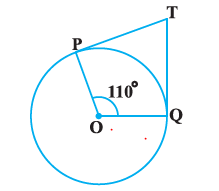Sol. ∵TQ and TP are tangents to a circle with centre O.
such that ∠POQ = 110°
∴ OP ⊥ PT and OQ ⊥ QT
⇒ ∠OPT = 90° and ∠OQT = 90°
Now, in the quadrilateral TPOQ, we get
∴ ∠PTQ + 90° + 110° + 90° = 360°
⇒ ∠PTQ + 290° = 360°
⇒ ∠PTQ = 360° – 290° = 70°
Thus, the correct option is (B).
Q.3.   Choose the correct option:
If tangents PA and PB from a point P to a circle with centre O are inclined to each other at angle of 80°, then ∠POA is equal to
(A) 50°
(B) 60°
(C) 70°
(D) 80°
Sol. Since, O is the centre of the circle and two tangents from P to the circle are PA and PB.
∴ OA ⊥ AP and OB ⊥ BP
⇒ ∠OAP = ∠OBP = 90°
Now, in quadrilateral PAOB, we have: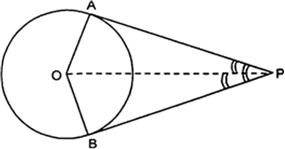∠APB + ∠PAO + ∠AOB + ∠PBO = 360°
⇒ 80° + 90° + ∠AOB + 90° = 360°
⇒ 260° + ∠AOB = 360°
⇒ ∠AOB = 360° – 260°
⇒ ∠AOB = 100°
In rt ΔOAP and rt ΔOBP, we have
OP = OP
∠OAF = ∠OBP
OA = OB
∴ Δ OAP ≌ Δ OBP
∴ Their corresponding parts are equal
⇒ ∠POA = ∠POBThus, the option (A) is correct.
Q.4.   Prove that the tangents drawn at the ends of a diameter of a circle are parallel.
Sol. In the figure, we have:
PQ is diameter of the given circle and O is its centre.
Let tangents AB and CD be drawn at the end points of the diameter PQ.
Since the tangent at a point to a circle is perpendicular to the radius through the point.
PQ ⊥ AB ⇒ ∠APQ = 90°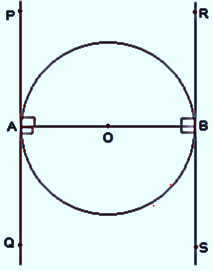And PQ ⊥ CD ⇒ ∠PQD = 90°
⇒ ∠APQ = ∠PQD
But they form a pair of alternate angles.
AB ∴∴ CD.
Q.5.   Prove that the perpendicular at the point of contact to the tangent to a circle passes through the centre.
Sol. In the figure, the centre of the circle is 0 and tangent AR touches the circle at P. If possible, let PQ be perpendicular to AB such that it is not passing through O.
Join OP.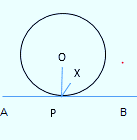Since tangent at a point to a circle is perpendicular to the radius through that point,
∴ AB ⊥ OP                i.e. ∠OPB = 90°                ...(1)
But by construction,
AB ⊥ PQ ⇒                ∠QPB = 90°                ...(2)
From (1) and (2),
∠QPB = ∠OPB
which is possible only when O and Q coincide.
Thus, the perpendicular at the point of contact to the tangent passes through the centre.
Q.6.   The length of a tangent from a point A at distance. 5 cm from the centre of the circle is 4 cm. Find the radius of the circle.
Sol. ∵The tangent to a circle is perpendicular to the radius through the point of contact.
∠OTA = 90°
Now, in the right ΔOTA, we have: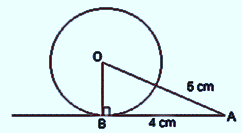OP2 = OT2 + PT2
⇒ 52 = OT2 + 42
⇒ OT2 = 52 – 42
⇒ OT2 = (5 – 4) (5 + 4)
⇒ OT2 = 1 × 9 = 9 = 32
⇒ OT = 3
Thus, the radius of the circle is 3 cm.
Q.7.   Two concentric circles are of radii 5 cm and 3 cm. Find the length of the chord of the larger circle which touches the smaller circle.
Sol. In the figure, O is the common centre, of the given concentric circles.
AB is a chord of the bigger circle such that it is a tangent to the smaller circle at P.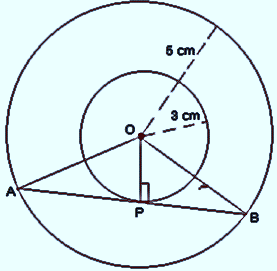Since OP is the radius of the smaller circle through P, the point of contact,
∴ OP ⊥ AB
⇒ ∠APB = 90°
Also, a radius perpendicular to a chord bisects the chord.Now, in right ΔAPO,
OA2 = AP2 – OP2
⇒ 52 = AP2 – 32
⇒ AP2 = 52 – 32
⇒ AP2 = (5 – 3) (5 + 3) = 2 × 8
⇒ AP2 = 16 = (4)2
⇒ AP = 4 cmHence, the required length of the chord AB is 8 cm.
Q.8.   A quadrilateral ABCD is drawn to circumscribe a circle (see figure). Prove that:
AB + CD = AD + BC
Sol. Since the sides of quadrilateral ABCD, i.e., AB, BC, CD and DA touch the circle at P, Q, R and S respectively, and the lengths of two tangents to a circle from an external point are equal.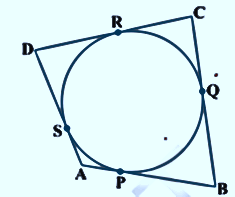AP = AS
BP = BQ
DR = DS
CR = CQ
(AP + BP) + (CR + RD) = (BQ + QC) + (DS + SA)
⇒ AB + CD = BC + DA
which was to be proved.
Q.9.   In the figure, XY and X'Y'are two parallel tangents to a circle with centre 0 and another tangent AB with point of contact C intersecting XY at A and XY' at B. Prove that ZAOB = W.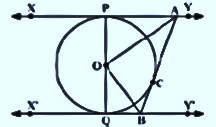Sol. ∵The tangents drawn to a circle from an external point are equal.
∴ AP = AC
In Δ PAO and Δ AOC, we have:
AO = AO                                          [Common]
OP = OC                                          [Radii of the same circle]
AP = AC
⇒ ΔPAO ≌ ΔAOC
∴ ∠PAO = ∠CAO
∠PAC = 2 ∠CAO                                                        ...(1)
Similarly ∠CBQ = 2 ∠CBO                                          ...(2)
Again, we know that sum of internal angles on the same side of a transversal is 180°.
∴ ∠PAC + ∠CBQ = 180°
⇒ 2∠CAO + 2 ∠CBO = 180°                                          [From (1) and (2)]⇒ 90° + ∠AOB = 180°
⇒ ∠AOB = 180° – 90°
⇒ LAOB = 90°.
Q.10.   Prove that the angle between the two tangents drawn from an external point to a circle is supplementary to the angle subtended by the line-segment joining the points of contact at the centre.
Sol. Here, let PA and PB be two tangents drawn from an external point P to a circle with centre O.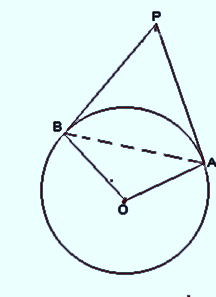Now, in right Δ OAP and right Δ OBP, we have
PA = PB                                             [Tangents to circle from an external point P]
OA = OB                                             [Radii of the same circle]
OP = OP                                             [Comm]
∴ By SSS congruency,
Δ OAP ≌ OBP
∴ Their corresponding parts are equal.
∠OAA = ∠OPB
And ∠AOP = ∠BOP
⇒ ∠APB = 2 ∠OPA and ∠AOS = 2 ∠AOP
But ∠AOP = 90° – LOPA
⇒ 2 ∠AOP = 180° – 2 ∠OPA
⇒ ∠AOB = 180° – ∠APB
⇒ ∠AOB + ∠APB = 180°.
Q.11.   Prove that the parallelogram circumscribing a circle is a rhombus. (CBSE 2012, CBSE Delhi 2014)
Sol. We have ABCD, a parallelogram which circumscribes a
circle (i.e., its sides touch the circle) with centre D.
Since tangents to a circle from an external point are equal in length
∴ AP = AS
BP = BQ
CR = CQ
DR = DS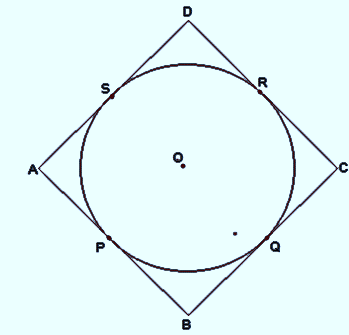(AP + BP) + (CR + DR) = (AS + DS) + (BQ + CQ)
⇒ AB + CD = AD + BC
But AB = CD                                                        [opposite sides of ABCD]
∴ AB + CD = AD + BC ⇒ 2 AB = 2 BC
⇒ AB = BC
Similarly AB = DA and DA = CD
Thus, AB = BC = CD = AD
Hence ABCD is a rhombus.
Q.12.   A triangle ABC is drawn to circumscribe a circle of radius 4 cm such that the segments BD and DC into which BC is divided by the point of contact D are of lengths 8 cm and 6 cm respectively (see figure). Find tlic sides AR and AC.
Sol. Here Δ ABC subscribe the circle with centre O.
∵ The sides BC, CA and AB touch the circle at D, E and F respectively.
∴ BF = BD = 8 cm
CE = CD = 6 cm
AF = AE = x cm (say)
⇒ The sides of the triangle are: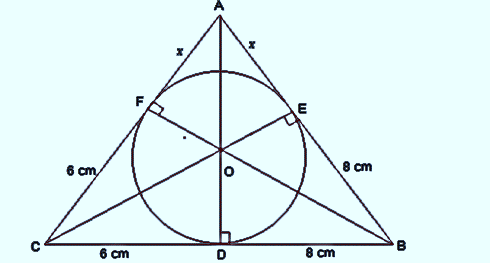14 cm, (x + 6) cm and (x + 8) cm
Perimeter of Δ ABC
= [14 + (x + 6) + (x + 8)] cm
= [14 + 6 + 8 + 2x] cm
= 28 + 2x cm
⇒ Semi perimeter of Δ ABC∴ S – AB = (14 + x) – (8 + x) = 6
S – BC = (14 + x) – (14) = x
S – AC = (14 + x) – (16 + x) = 8∴ ar (ΔABC) = ar (Δ OBC) + ar (Δ OCA) + ar (Δ OAB)
= 28 cm2 + (2x + 12) cm2 + (2x + 16) cm2
= (28 + 12 + 16) + 4x cm2
= (56 + 4x) cm2
From (1) and (2), we have:Squaring both sides
(14 + x)2 = (14 + x) 3x
⇒ 196 + x2 + 28x = 45x + 3x2
⇒ 2x2 + 14x – 196 = 0 ⇒ x2 + 7x – 98 = 0
⇒ (x – 7) (x + 14) = 0
⇒ Either x – 7 = 0 ⇒ x = 7
or x +14 = 0 ⇒ x = (–14)
But x = (– 14) is not required
∴ x = 7 cm
Thus, AB = 8 + 7 = 15 cm
BC = 8 + 6 = 14 cm
CA = 6 + 7 = 13 cm.
Q.13.   Prove that opposite sides of a quadrilateral circumscribing a circle subtend supplementary angles at the centre of the circle.
Sol. We have a circle with centre O.
A quadrilateral ABCD is such that the sides AB, BC, CD and DA touch the circle at P, Q,,F R and S respectively.
Let us join OP, OQ, OR and OS. We know that two tangents drawn from an external point to a circle subtend equal angles at the centre.
∴ ∠1 = ∠2
∠3 = ∠4
∠5 = ∠6 and ∠7 = ∠8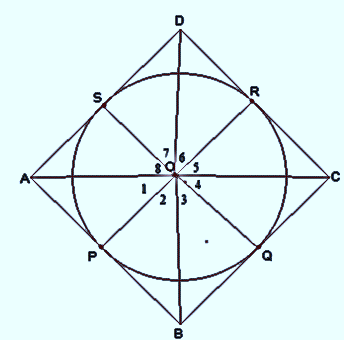Also, the sum of all the angles around a point is 360°.
∴ ∠1 + ∠2 + ∠3 + ∠4 + ∠5 + ∠6 + ∠7 + ∠8 = 360°
∴ 2 [∠1 + ∠8 + ∠5 + ∠4] = 360°
⇒ (∠1 + ∠8 + ∠5 + ∠4) = 180° (1)
And 2 [∠2 + ∠3 + ∠6 + ∠7] = 360°
⇒ (∠2 + ∠3) + (∠6 + ∠7) = 180° (2)
Since, ∠2 + ∠3 = ∠AOB
∠6 + ∠7 = ∠COD
∠1 + ∠8 = ∠AOD
∠4 + ∠5 = ∠BOC
∴ From (1) and (2), we have:
∠AOD + ∠BOC = 180° and
∠AOB + ∠COD = 180°